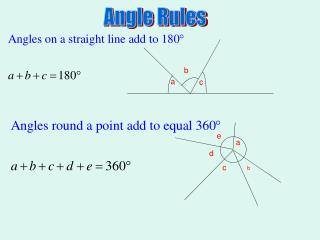Download PresentationAngle Rules

# Angle Rules - PowerPoint PPT PresentationDownload Presentation## Angle Rules

- - - - - - - - - - - - - - - - - - - - - - - - - - - E N D - - - - - - - - - - - - - - - - - - - - - - - - - - -
##### Presentation Transcript

1. b a c e a d c b Angle Rules

2. b a c c b a d

3. b a c d Complementary Angles add to 90o The complement of 55o is 35o because these add to 90o Supplementary Angles add to 180o The supplement of 55o is 125o because these add to 180o C before S 90 before 180

4. *

5. *

6. *

7. Rules of Parallel Lines

8. x

9. a

10. a

11. 1 180÷3=60 360 180 180×2 =360 360 2 360÷4=90 180×3 =540 3 360 540÷5=108 180×4 =720 720÷6=120 4 360 360 180×10 =1800 1800÷12=150 10 360

12. For ANY polygon For regular polygons only For regular polygons only

13. 155 135 130 x 95 x 80 135 130 95 135 Degrees in the polygon: Degrees in the polygon :

14. This is a regular Polygon

15. Similar Triangles

16. Similar Triangles If triangles are similar: Corresponding side lengths are in proportion. (One triangle is an enlargement of the other) Corresponding angles in the triangle are the same 25m 20m x 4 m It doesn’t matter which way round you make the fraction BUT you must do the same for both sides It is sensible to start with the x so it is on the top

17. If the angles of two triangles are the same, they are similar triangles.

18. #11 x x x x x

19. Lesson 6 Circle Language and Angle at Centre

20. Equal Radii: Two radii in a circle always form an isosceles triangle Isos , = radii

21. x Base ‘s isos Δ, = radii Base ‘s isos Δ, = radii Sum of Δ = 180° *

22. Angle at the centre is twice the angle at the circumference a a 2a 2a 2a 2a a a

23. Lesson 7 Tangent is perpendicular to the radius and Angles on Same Arc are equal

24. Tangent is perpendicular to the radius

25. Angles on the same arc are equal ‘s On the same arch equal

26. *

27. *

28. *

30. Cyclic Quadrilaterals A quadrilateral which has all four vertices on the circumference of a circle is called a Cyclic quadrilateral Rule 1:

31. Rule 2: The exterior angle of a cyclic quadrilateral is equal to the opposite interior angle

32. *

33. *

34. Tangents When two tangents are drawn from a point to a circle, they are the same length

35. *

36. Similar Triangles If triangles are similar: Corresponding side lengths are in proportion. (One triangle is an enlargement of the other) Corresponding angles in the triangle are the same 25m 20m x 4 m It doesn’t matter which way round you make the fraction BUT you must do the same for both sides It is sensible to start with the x so it is on the top

37. If the angles of two triangles are the same, they are similar triangles.